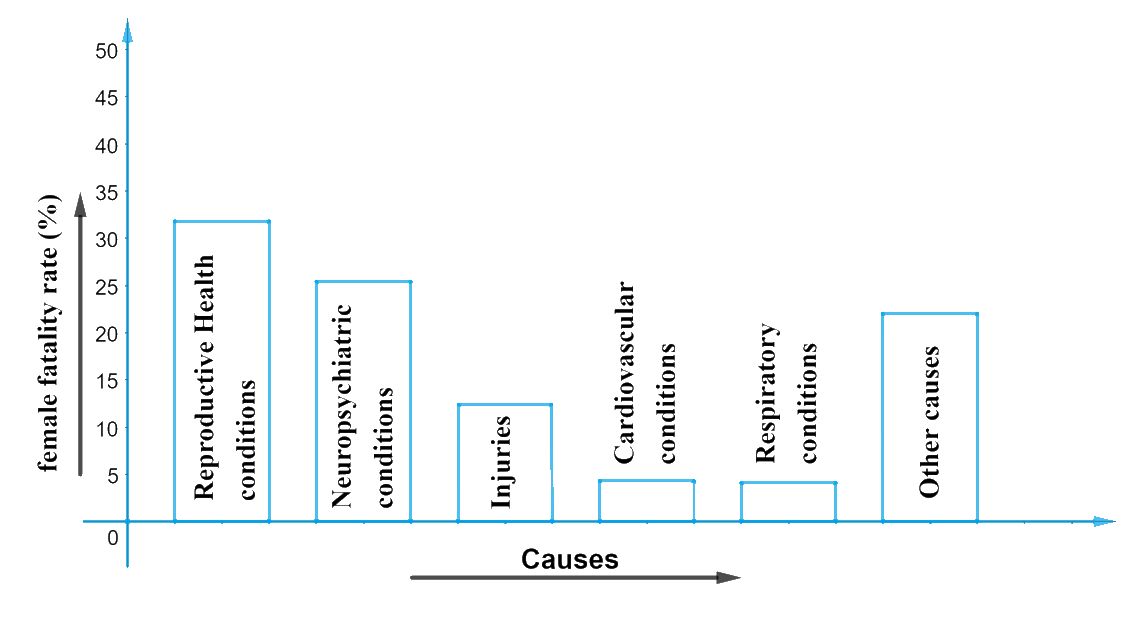# Ex.14.3 Q1 Statistics Solution - NCERT Maths Class 9

Go back to  'Ex.14.3'

## Question

A survey conducted by an organization for the cause of illness and death among the women between the ages $$15 - 44$$ (in years) worldwide, found the following figures (in $$\%$$):

 S. No. Causes Female fatality rate (%) $$1.$$ Reproductive health conditions $$31.8$$ $$2.$$ Neuropsychiatric conditions $$25.4$$ $$3.$$ Injuries $$12.4$$ $$4.$$ Cardiovascular conditions $$4.3$$ $$5.$$ Respiratory conditions $$4.1$$ $$6.$$ Other causes $$22.0$$
1. Represent the information given above graphically.
2. Which condition is the major cause of women’s ill health and death worldwide?
3. Try to find out, with the help of your teacher, any two factors which play a major role in the cause in (ii) above being the major cause.

Video Solution
Statistics
Ex exercise-14-3 | Question 1

## Text Solution

What is known?

Female fatality rate($$\%$$)

What is Unknown?

1. Represent the given information graphically
2. Major cause of women’s ill health and death worldwide.
3. Factors that play a major role in the major cause of women’s illness and death.

Reasoning:

The above data can be represented graphically using a bar graph as below

1. We will represent the causes on the $$x$$-axis, maintaining equal widths for all bars and with equal gaps in between. One cause will be represented by one unit.
2. We will represent the fatality rate on the y-axis with a scale of unit as $$5\%$$ as the max value.It can be visualized from the graph that

1. Fatality rate due to reproductive health condition is more than double that of fatality rate owing to ‘injuries. Reproductive health condition can be considered as the major cause of women’s ill health worldwide.
2. The factors contributing to (i) can be as follows:
(a) Lack of awareness / knowledge about reproductive health conditions.
(b) Lack of emergency medical facilities.